Next: Algorithm Up: DINSAR Previous: Output Description   Contents

# Implementation

Simple equations for topo-pair (no deformation, no atmosphere, no other errors,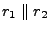)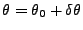(1)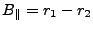(2)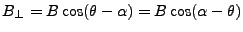(3)(4)

The baseline components, for points on the reference ellipsoid (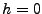) are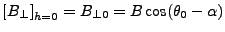(5)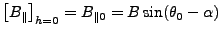(6)

The 'true' phase of the interferogram is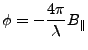(7)

And corrected for the phase of the reference body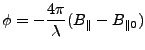(8)

For the defo-pair (1,3), denoted with a prime, similar equations follow. Deformation in the line of sight (range), that occurred in between the acquisitions, is denoted by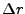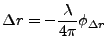(9)

A positiveimplies deformation in the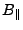direction (away from the sensor, i.e., subsidence). The phase of this interferogram is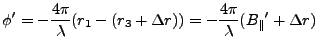(10)

Combining the expressions for the interferometric phase for the topo-pair (31.7) and defo-pair (31.10) yields: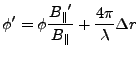(11)

The problem here is that the 'true' parallel baselines are unknown.

The (actually wrapped) phase of the deformation interferogram, corrected for reference phase, is defined as: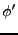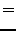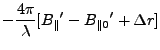(12)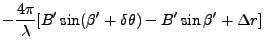where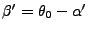. Using the approximation for small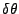(which is about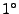or 0.0175 rad for terrain height differences of 5 km)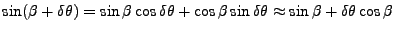(13)

it follows from equation 31.13 that the 'flat earth' corrected phase equals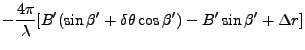(14)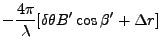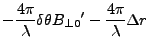The corrected phase for the topo pair equals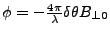(using the same approximation), and combining this with 31.15 yields (for the 'flat earth' corrected phases)(15)

or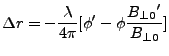(16)

or for the phase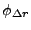caused by the deformation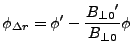(17)

This important equation shows how to obtain offset vectors from 3 SLC images, i.e., by scaling the (reference phase corrected) unwrapped phase of the topo-pair by the ratio of the perpendicular baselines (to points on reference body), and subtracting this from the phase of the defo-pair. This can thus be performed without the 'true' values for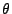are required.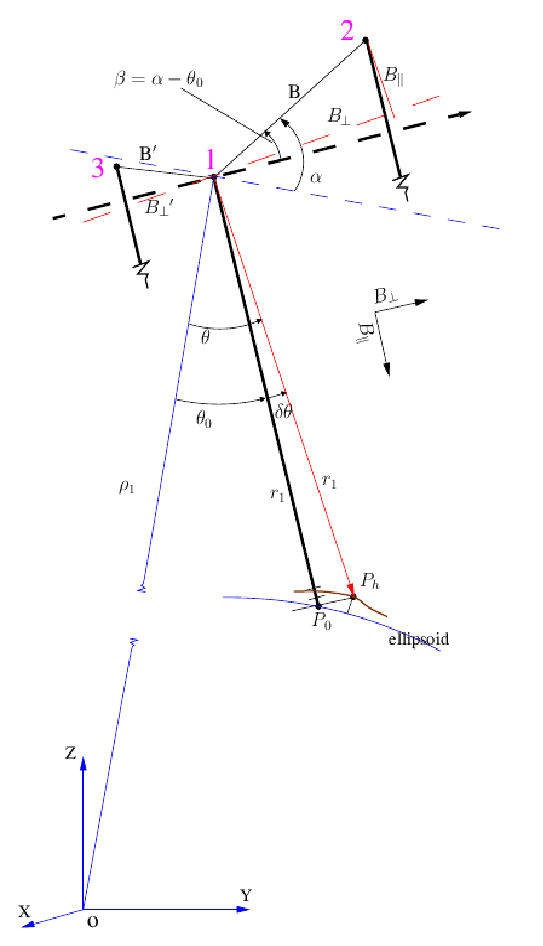SubsectionsNext: Algorithm Up: DINSAR Previous: Output Description   Contents
Leijen 2009-04-14